## CAM是什幺？

CAM全称Class Activation Mapping，既类别激活映射图，也被称为类别热力图、显着性图等。是一张和原始图片等同大小图，该图片上每个位置的像素取值范围从0到1，一般用0到255的灰度图表示。可以理解为对预测输出的贡献分布，分数越高的地方表示原始图片对应区域对网络的响应越高、贡献越大。

```img_color = cv2.applyColorMap(img_gray, cv2.COLORMAP_JET) # 将灰度图转化为伪色彩图，
# COLORMAP_JET 为输出的颜色模式```A.原始图片 B. CAM灰度图 C. CAM彩色热图 D. 原图+CAM热图叠加

## CAM有什幺用？

CAM主要有以下作用:A.原始图片 B. Resnet50 预测结果 C. Resnet34 预测结果## 如何获取CAM？step1：提取需要可视化的特征层，例如尺寸为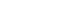的张量；

step2：获取该张量的每个channel的权重，即长度为512的向量；

step3：通过线性融合的方式，将该张量在channel维度上加权求和，获取尺寸为7*7的map；

step4：对该map进行归一化，并通过插值的方式resize到原图尺寸；

CAM (2015.12)

score-CAM (2019.10)

ss-CAM (2020.06)

Ablation-CAM (2020)

## 开山之作：利用GAP获取CAMGAP的好处是：

GAP直接对特征层的空间信息进行求和，整合了整个空间的信息，所以网络对输入的空间变化的鲁棒性更强。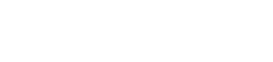#### 核心代码解读:

```# 获取全连接层的权重
self._fc_weights = self.model._modules.get(fc_layer).weight.data
# 获取目标类别的权重作为特征权重
weights=self._fc_weights[class_idx, :]
# 这里self.hook_a为最后一层特征图的输出
batch_cams = (weights.unsqueeze(-1).unsqueeze(-1) * self.hook_a.squeeze(0)).sum(dim=0)
# relu操作,去除负值
batch_cams = F.relu(batch_cams, inplace=True)
# 归一化操作
batch_cams = self._normalize(batch_cams)```2.CAM只能提取最后一层特征图的热力图，而gard-CAM可以提取任意一层；

B. 目标类别score 是用 通过softmax之后的还是之前的？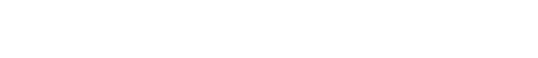#### 核心代码解读:

```# 利用onehot的形式锁定目标类别
one_hot = np.zeros((1, output.size()[-1]), dtype=np.float32)
one_hot[index] = 1
# 获取目标类别的输出,该值带有梯度链接关系,可进行求导操作
one_hot = torch.sum(one_hot * output)
one_hot.backward(retain_graph=True) # backward 求导
# 获取对应特征层的梯度map
target = features[-1].cpu().data.numpy()[0, :] # 获取目标特征输出
weights = np.mean(grads_val, axis=(2, 3))[0, :] # 利用GAP操作, 获取特征权重
cam = weights.dot(target.reshape((nc, h * w)))
# relu操作,去除负值, 并缩放到原图尺寸
cam = np.maximum(cam, 0)
cam = cv2.resize(cam, input.shape[2:])
# 归一化操作
batch_cams = self._normalize(batch_cams)```#### 核心代码解读:

```## 获取特征权重的过程
# 反向传播
self._backprop(scores, class_idx)
# 注意,这里用乘法,因为论文中将2次偏导和3次偏导进行了2次方和3次方的转化
# 获取alpha权重map
# 利用alpha 权重map去获取特征权重
return alpha.squeeze_(0).mul_(torch.relu(self.hook_g.squeeze(0))).sum(axis=(1, 2))```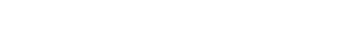n为对原图增加噪声并前向的次数，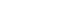为高斯噪声。可以看到，随着n的增加，噪声逐渐减少，saliency maps逐渐聚焦到目标物体区域。#### 核心代码解读:

```for i in range(self.n_samples): # 进行n_samples次加噪声操作
# 输入图片增加高斯噪声
score = self.model(x_with_noise)
score[0, idx].backward(retain_graph=True) # 求梯度
activations = self.values.activations
n, c, _, _ = gradients.shape
denominator += \
ag.view(n, c, -1).sum(-1, keepdim=True).view(n, c, 1, 1)
denominator = torch.where(
denominator != 0.0, denominator, torch.ones_like(denominator))
alpha = numerator / (denominator + 1e-7)
# 获取weights
weights = (alpha * relu_grad).view(n, c, -1).sum(-1).view(n, c, 1, 1)
# 对特征层加权融合, 并进行relu+归一化操作
cam = (weights * activations).sum(1, keepdim=True)
cam = F.relu(cam)
cam -= torch.min(cam)
cam /= torch.max(cam)
total_cams += cam
total_cams /= self.n_samples # 求平均操作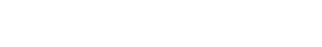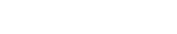#### 核心代码解读:

```with torch.no_grad():# gradient-free, 所以不需要计算梯度
for i in range(K): # 对K层特征图进行遍历操作
# 获取第i层特征图,并进行上采样操作
saliency_map = torch.unsqueeze(activations[:, i, :, :], 1)
saliency_map = F.interpolate(saliency_map, size=(h, w), mode='bilinear')
# 归一化
norm_saliency_map = (saliency_map - saliency_map.min()) /
(saliency_map.max() - saliency_map.min())
output = self.model_arch(input * norm_saliency_map)
output = F.softmax(output)
score = output[predicted_class]
# 利用该得分作为权重对该层的特征图进行加权线性融合, baseline默认为全0的图,所以这里直接
# 用该得分作为特征权重
score_saliency_map +=  score * saliency_map
# relu去除负值
score_saliency_map = F.relu(score_saliency_map)
# 归一化
score_saliency_map = (score_saliency_map - score_saliency_map.min())/
(score_saliency_map_max - score_saliency_map.max())
# score_saliency_map 为所求
return score_saliency_map```## 利用Ablation分析的方法：Ablation-CAMk为特征图的channel个数，这里的是原始图片c类别的类别score输出， 为将特征图第k个channel全部设置为0后，将原始图片再送进网络，获取的c类别的score。二者得分的之差，再除以就获取slope，其实就是特征融合的权重。